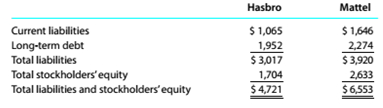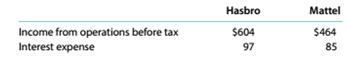Chapter 9, Problem 9.14E

Chapter
Section
Textbook Problem

Debt ratio, ratio of liabilities to stockholders' equity, and times interest earned Hasbro (HAS) and Mattel. Inc. (MAT), are the two largest toy companies in North America, liability and stockholders' equity data from recent balance sheets are shown for each company below (in millions):The income from operations and interest expense from the income statement for both companies were as follows (in millions):a.Determine the debt ratio for both companies. Round lo one decimal place. b. Determine the ratio of liabilities to stockholders' equity for In both companies. Round to one decimal place. c. Determine the times interest earned for both companies. Round to one decimal place. d. Interpret the ratio differences between the two companies.

To determine

(a)

Introduction:

Accounting ratios are used to evaluate the financial performance of the business organisation

Debit ratio:

It measures the extents of company's leverage. It can be interpreted as proportion of company's assets financed by debts. It can be calculated by

Debit ratio=total debt total assets

To calculate:

Debit ratio of both the companies.

Explanation
 Particulars H M Total Liabilities $3,017$3,920 Total Assets $4,721$6,553
To determine

(b)

Concept Introduction:

Ratio of liabilities to shareholders' equity or debt to equity ratio: is used to evaluate company's financial leverage, it reflects the ability of shareholders equity to cover all outstanding debts.it can be calculated as follows.

Debt to equity ratio= total debttotal shareholders equity

To calculate:

Debt to equity ratio both the companies.

To determine

(c)

Concept Introduction:

Time interest earned TIE :

It is a matric used to measure a company's ability to meet debit obligation it can be calculated using following formula.

Time interest earned=Earning before interest and taxes  Total Interest payable

To calculate:

Time interest earned for both the companies.

To determine

(d)

Introduction:

Accounting ratios are used to evaluate the financial performance of the business organisation

Solvency analysis:

Solvency analysis is used to evaluate companies' ability to pay its long-term debt, it also helps owner to determine the chances of firm's long-term survival, some of the ratios used for solvency analysis are as follows

To Interpret:

Ratio difference between both the companies.

Still sussing out bartleby?

Check out a sample textbook solution.

See a sample solution

The Solution to Your Study Problems

Bartleby provides explanations to thousands of textbook problems written by our experts, many with advanced degrees!

Get Started

What is a LAN?

Accounting Information Systems

How does the journal differ from the ledger?

College Accounting (Book Only): A Career Approach

What are control limits, and how are they set?

Managerial Accounting: The Cornerstone of Business Decision-Making

What is inflation and what causes it?

Principles of Microeconomics (MindTap Course List)

EXCHANGE RATES Use the foreign exchange section of a current issue of The Watt Street Journal to look up the si...

Fundamentals of Financial Management, Concise Edition (with Thomson ONE - Business School Edition, 1 term (6 months) Printed Access Card) (MindTap Course List)

What is a biological asset?

Corporate Financial Accounting